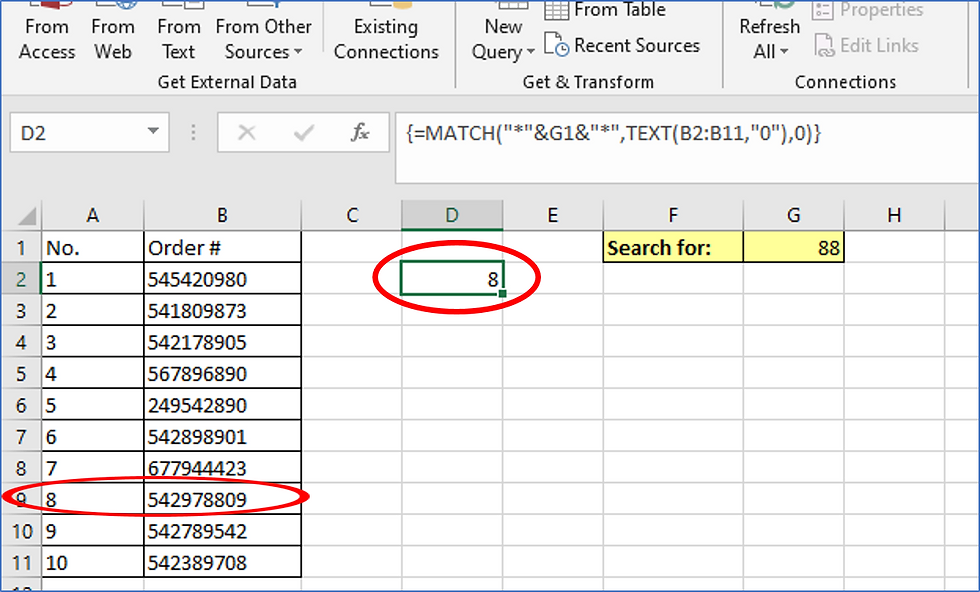top of pageSearch

# Wildcard search for a string of numbers

You can use a MATCH formula to find where a string of numbers appears inside any one of a long list of numbers. The array formula will return the number of the row in an array where the first instance of the string appears.

In this example, we search for the number string in cell G1, by adding wildcard asterisks before and after it in the MATCH formula. Then we enter the range to search in followed by zero in the nested TEXT formula. The TEXT formula converts the numbers to text, otherwise the wildcard search could not be run on the numbers.This is an array formula so be sure to press CTRL + SHIFT when entering it in the cell. The formula returns the number of the row in the range where the searched for string, 88, appears the first time - the long order number - 542978809.bottom of page